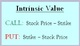# How to calculate intrinsic value of stock options in the share market?

By Research DeskIntrinsic value, in context of option trading, is the amount by which the strike price of an option is in the money.

Call Option Intrinsic Value = Current Stock Price – Call Strike Price.

If the above value is positive, then the option is ‘In the money’. If it is negative, then the option is ‘out of the money’ and if it zero, it is ‘at the money’

Put Option Intrinsic Value = Put Strike Price - Current Stock Price

If the above value is positive, then the option is ‘Out of the money’. If it is negative, then the option is ‘In the money’ and if it zero, it is ‘at the money’

Intrinsic value is the difference between the underlying price and the strike price, to the extent that this is in favor of the option holder. In simple words, it is the value which is already available in the market.

Take for example, if you are holding NIFTY 8,500 Call (Long) option and NIFTY is at 8,550 level then you already have Rs. 50 advantage even if the option expires today. This Rs. 50 is the intrinsic value of the 8,500 Call option.

Conversely if you are holding a put option and NIFTY is below strike price then your option has an intrinsic value with is equal to the difference between the strike price and NIFTY value.